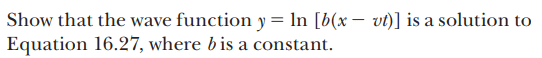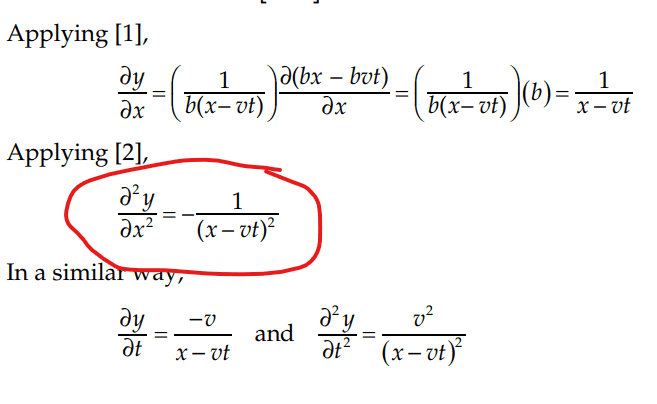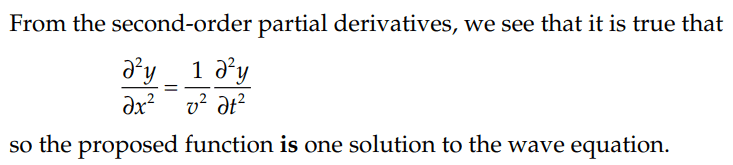# Showing that this equation is a solution to the linear wave equation

ChiralSuperfields
Homework Statement:
Relevant Equations:
For this problem,Where equation 16.27 is the wave equation.

The solution isI don't understand how they got the second partial derivative of ##y## with respect to
##x## circled in red.

I thought it would be ##1## since ##v## and ##t## are constants

Many thanks!

Gold Member
MHB

For this problem,
View attachment 321932
Where equation 16.27 is the wave equation.

The solution is
View attachment 321933
View attachment 321934

I don't understand how they got the second partial derivative of ##y## with respect to
##x## circled in red.

I thought it would be ##1## since ##v## and ##t## are constants

Many thanks!
You are likely over-thinking this. Use the chain rule.

Big hint:
##\dfrac{ \partial }{ \partial x} \dfrac{1}{x - vt} \rightarrow \dfrac{ \partial }{ \partial u } \dfrac{1}{u} \cdot \dfrac{ \partial u}{ \partial x}##

What would you use for u?

-Dan

•ChiralSuperfields
ChiralSuperfields
You are likely over-thinking this. Use the chain rule.

Big hint:
##\dfrac{ \partial }{ \partial x} \dfrac{1}{x - vt} \rightarrow \dfrac{ \partial }{ \partial u } \dfrac{1}{u} \cdot \dfrac{ \partial u}{ \partial x}##

What would you use for u?

-Dan

##u = x- vt##

Sorry do you please know why we need to use the chain rule?

Thank you!

Homework Helper
Gold Member
2022 Award
why we need to use the chain rule?
Because that is what tells you how to differentiate ##\frac 1{x-vt}## wrt ##x##. First, you differentiate it wrt ##x-vt##, 'cos that's easy, then you multiply by the derivative of ##x-vt## wrt ##x##.

•ChiralSuperfields and topsquark
Homework Helper
Gold Member
2022 Award
Isn't ##y = f(x - vt)## a solution to the wave equation, for any twice differentiable ##f##?

•ChiralSuperfields, topsquark and haruspex
Staff Emeritus
Homework Helper
I thought it would be ##1## since ##v## and ##t## are constants
Perhaps you "misspoke" here? ##t## is the time variable, as you must be aware?

•ChiralSuperfields and topsquark
ChiralSuperfields
Because that is what tells you how to differentiate ##\frac 1{x-vt}## wrt ##x##. First, you differentiate it wrt ##x-vt##, 'cos that's easy, then you multiply by the derivative of ##x-vt## wrt ##x##.

Sorry could you please explain a bit more why we need to use the chain rule? I have not done partial derivatives from multivariate calculus yet.

Many thanks!

ChiralSuperfields
Isn't ##y = f(x - vt)## a solution to the wave equation, for any twice differentiable ##f##?

ChiralSuperfields
Perhaps you "misspoke" here? ##t## is the time variable, as you must be aware?

Yeah I agree that ##t## is a time variable, however, I thought I was meant to treat it has a constant along with ##v## since we are partially differentiating ##y## with respect to ##x##.

Many thanks!

Staff Emeritus
Homework Helper
Yeah I agree that ##t## is a time variable, however, I thought I was meant to treat it has a constant along with ##v## since we are partially differentiating ##y## with respect to ##x##.
Ah, okay. Yes, for the purposes of doing partial derivatives w.r.t. other variables, that's right.

•ChiralSuperfields
ChiralSuperfields
Ah, okay. Yes, for the purposes of doing partial derivatives w.r.t. other variables, that's right.

ChiralSuperfields
Because that is what tells you how to differentiate ##\frac 1{x-vt}## wrt ##x##. First, you differentiate it wrt ##x-vt##, 'cos that's easy, then you multiply by the derivative of ##x-vt## wrt ##x##.
I guess one way of thinking about it is thinking that ##x -vt## is in brackets to the power of 1 since you must always use chain rule for brackets. I guess we also have to use chain rule here because we can't bring the x up because it is with the other terms.

Let me know if I am correct!

Many thanks!

Homework Helper
Gold Member
2022 Award
I guess one way of thinking about it is thinking that ##x -vt## is in brackets to the power of 1 since you must always use chain rule for brackets. I guess we also have to use chain rule here because we can't bring the x up because it is with the other terms.

Let me know if I am correct!

Many thanks!
$$\frac d {dx}\bigg (\frac 1 {f(x)}\bigg ) = -\frac {f'(x)}{(f(x))^2}$$

•ChiralSuperfields
ChiralSuperfields
$$\frac d {dx}\bigg (\frac 1 {f(x)}\bigg ) = -\frac {f'(x)}{(f(x))^2}$$

Staff Emeritus
Homework Helper
I guess one way of thinking about it is thinking that ##x -vt## is in brackets to the power of 1 since you must always use chain rule for brackets.
You don't need the chain rule for
$$\frac{d}{dx}(x^2+x).$$ Parentheses, brackets, etc. don't automatically mean the chain rule.

Based on what you initially wrote, you seemed to be thinking
$$\frac{\partial}{\partial x} \frac{1}{x-vt} =\frac{1}{\frac{\partial}{\partial x}{(x-vt)}} = \frac 11 = 1.$$ I hope you see that the first equality is wrong. That's not how differentiation works.

Because you're differentiating a quotient, you could use the quotient rule and not use the chain rule. Most people, however, would use the chain rule because the numerator is a constant. It might help to rewrite the problem as
$$\frac{\partial}{\partial x} (x-vt)^{-1}.$$

I guess we also have to use chain rule here because we can't bring the x up because it is with the other terms.
Right.

•ChiralSuperfields and PeroK
ChiralSuperfields
You don't need the chain rule for
$$\frac{d}{dx}(x^2+x).$$ Parentheses, brackets, etc. don't automatically mean the chain rule.

Based on what you initially wrote, you seemed to be thinking
$$\frac{\partial}{\partial x} \frac{1}{x-vt} =\frac{1}{\frac{\partial}{\partial x}{(x-vt)}} = \frac 11 = 1.$$ I hope you see that the first equality is wrong. That's not how differentiation works.

Because you're differentiating a quotient, you could use the quotient rule and not use the chain rule. Most people, however, would use the chain rule because the numerator is a constant. It might help to rewrite the problem as
$$\frac{\partial}{\partial x} (x-vt)^{-1}.$$

Right.